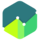COURSE

## Common Probability DistributionsThis course introduces some of the discrete and continuous probability distributions most commonly used to describe the behavior of random variables.

• Discrete and continuous random variables
• Probability distribution function and cumulative distribution function
• Discrete uniform and binomial distribution functions
• Using binomial trees to describe stock price movement
• Tracking error
• Continuous uniform distribution
• Normal distribution
• Univariate and multi-variate distributions
• Standard normal distribution
• Short-fall risk and safety-first ratio
• Normal vs. lognormal distribution
• Discrete and continuously compounded rates of return
• Monte Carlo simulation and historical simulation

#### Course Resources

Study Notes for Common Probability Distributions
Member-only

#### Course Quizzes